21 方法内联（下）

abstract class BinaryOp {
public abstract int apply(int a, int b);
}

public int apply(int a, int b) {
return a + b;
}
}

class Sub extends BinaryOp {
public int apply(int a, int b) {
return a - b;
}
}

基于类型推导的完全去虚化

public static int foo() {
return op.apply(2, 1);
}

public static int bar(BinaryOp op) {
return op.apply(2, 1);
}

foo 方法的 IR 图（方法内联前）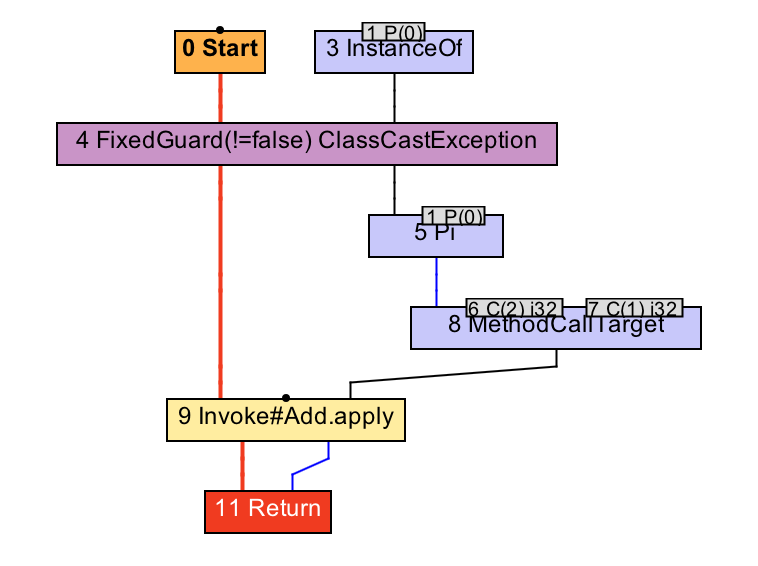bar 方法的 IR 图（方法内联前）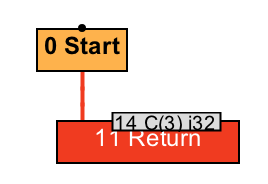foo 方法的 IR 图（方法内联及逃逸分析后）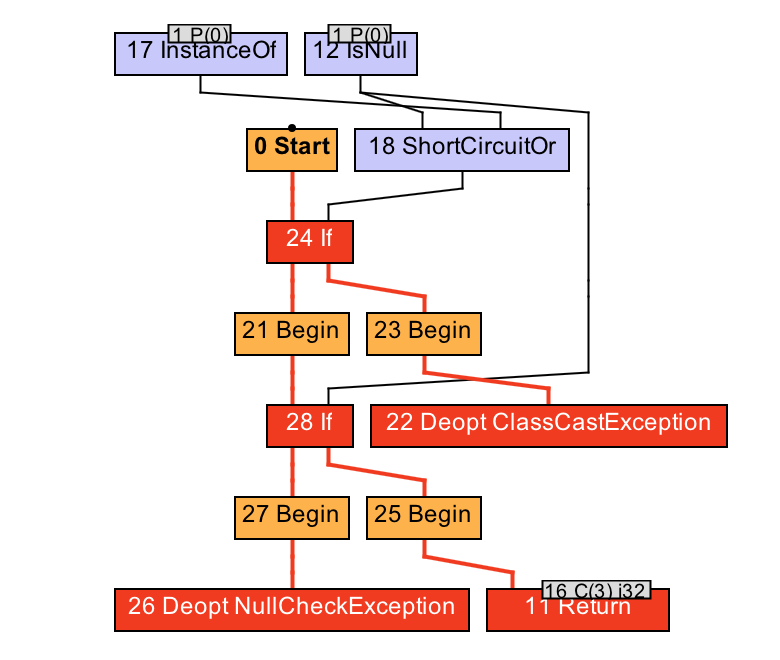bar 方法的 IR 图（方法内联后）

public static int notInlined(BinaryOp op) {
return op.apply(2, 1);
}
return 0;
}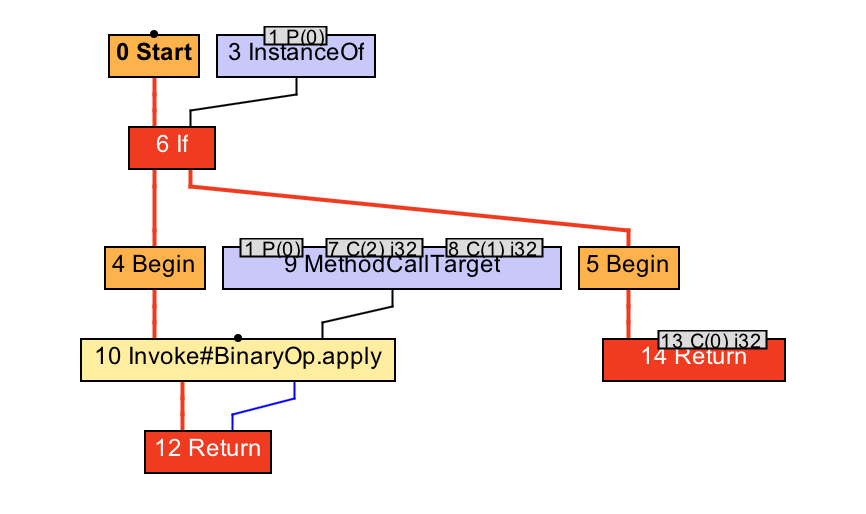基于类层次分析的完全去虚化

Java 虚拟机的做法是为当前编译结果注册若干个假设（assumption），假定某抽象类只有一个子类，或者某抽象方法只有一个具体实现，又或者某类没有子类等。

public static int test(BinaryOp op) {
return op.apply(2, 1);
}

public static int test(Add op) {
return op.apply(2, 1); // 仍需添加假设
}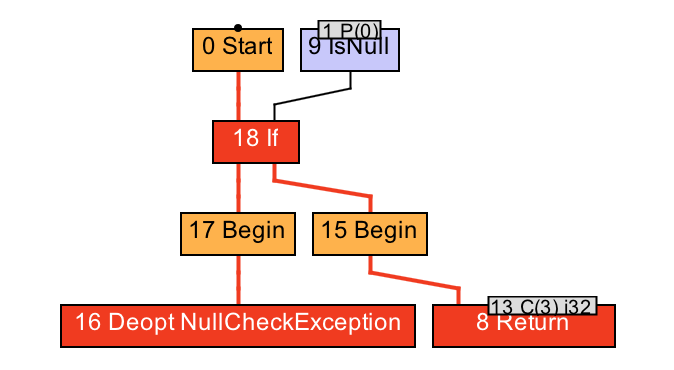test 方法的 IR 图（方法内联后）

Java 类验证器将接口类型直接看成 Object 类型，所以有可能出现声明类型为接口，实际类型没有继承该接口的情况，如下例所示。

// A.java
interface I {}

public class A {
public static void test(I obj) {
System.out.println("Hello World");
}

public static void main(String[] args) {
test(new B());
}
}

// B.java
public class B implements I { }

// Step 1: compile A.java and B.java
// Step 2: remove "implements I" from B.java, and compile B.java
// Step 3: run A

条件去虚化

public static int test(BinaryOp op) {
return op.apply(2, 1);
}

public static int test(BinaryOp op) {
if (op.getClass() == Sub.class) {
return 2 - 1; // inlined Sub.apply
} else if (op.getClass() == Add.class) {
return 2 + 1; // inlined Add.apply
} else {
... // 当匹配不到类型 Profile 中的类型怎么办？
}
}

当匹配不到动态类型时进行去优化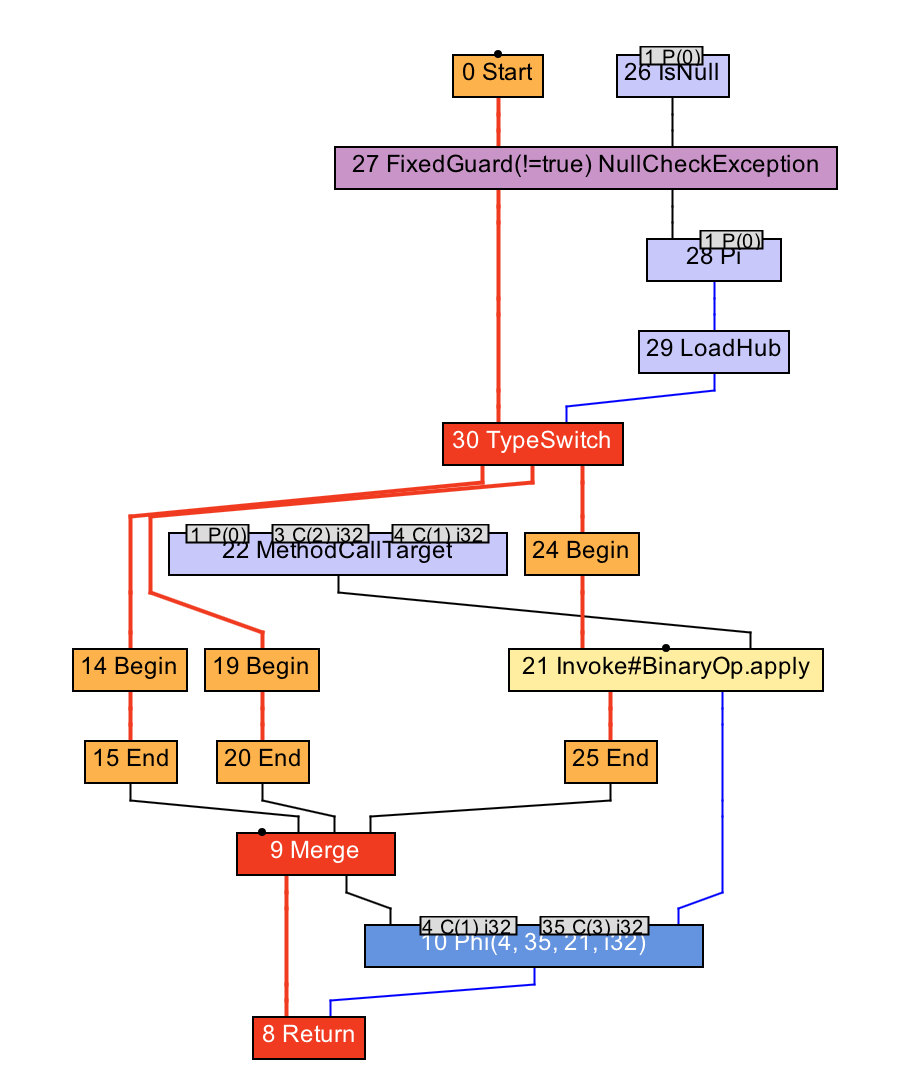总结与实践

// Run with java -XX:CompileCommand='dontinline JITTest.test' -XX:+PrintCompilation JITTest
public class JITTest {
static abstract class BinaryOp {
public abstract int apply(int a, int b);
}

static class Add extends BinaryOp {
public int apply(int a, int b) {
return a + b;
}
}

static class Sub extends BinaryOp {
public int apply(int a, int b) {
return a - b;
}
}

public static int test(BinaryOp op) {
return op.apply(2, 1);
}

public static void main(String[] args) throws Exception {